Скачать презентацию COMP 578 Genetic Algorithms for Data Mining Keith

d21f2eafc1808dcdbc62aea0a475b446.ppt

• Количество слайдов: 28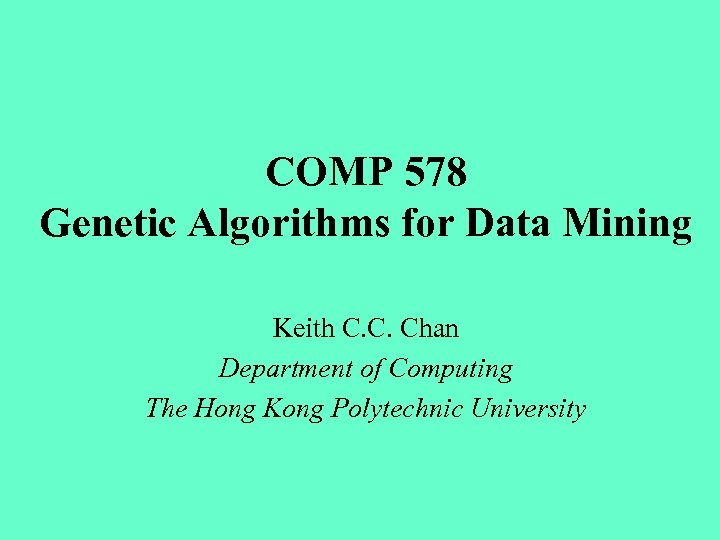COMP 578 Genetic Algorithms for Data Mining Keith C. C. Chan Department of Computing The Hong Kong Polytechnic University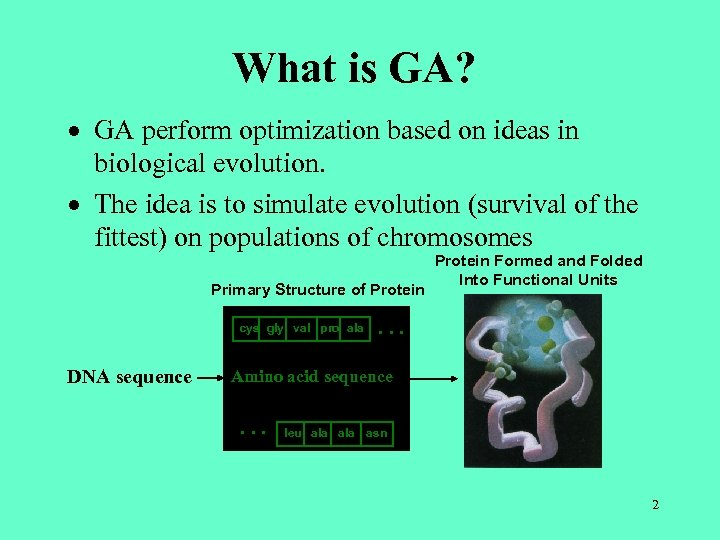What is GA? · GA perform optimization based on ideas in biological evolution. · The idea is to simulate evolution (survival of the fittest) on populations of chromosomes Primary Structure of Protein cys gly val pro ala DNA sequence Protein Formed and Folded Into Functional Units … Amino acid sequence … leu ala asn 2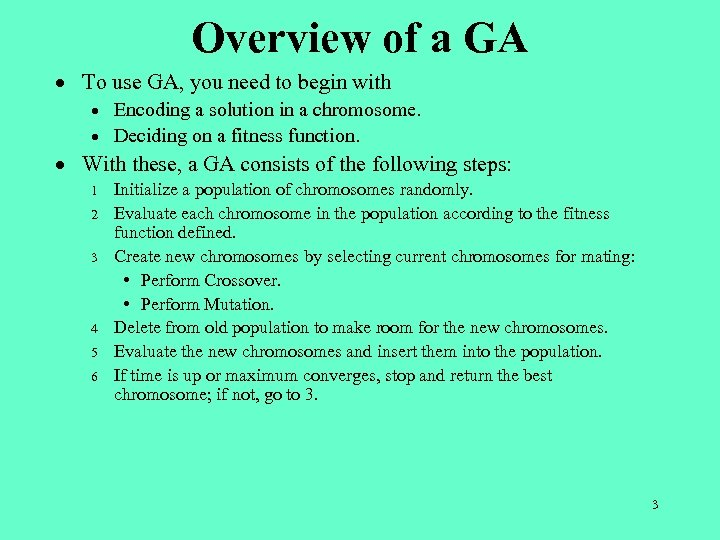Overview of a GA · To use GA, you need to begin with · · Encoding a solution in a chromosome. Deciding on a fitness function. · With these, a GA consists of the following steps: 1 2 3 4 5 6 Initialize a population of chromosomes randomly. Evaluate each chromosome in the population according to the fitness function defined. Create new chromosomes by selecting current chromosomes for mating: • Perform Crossover. • Perform Mutation. Delete from old population to make room for the new chromosomes. Evaluate the new chromosomes and insert them into the population. If time is up or maximum converges, stop and return the best chromosome; if not, go to 3. 3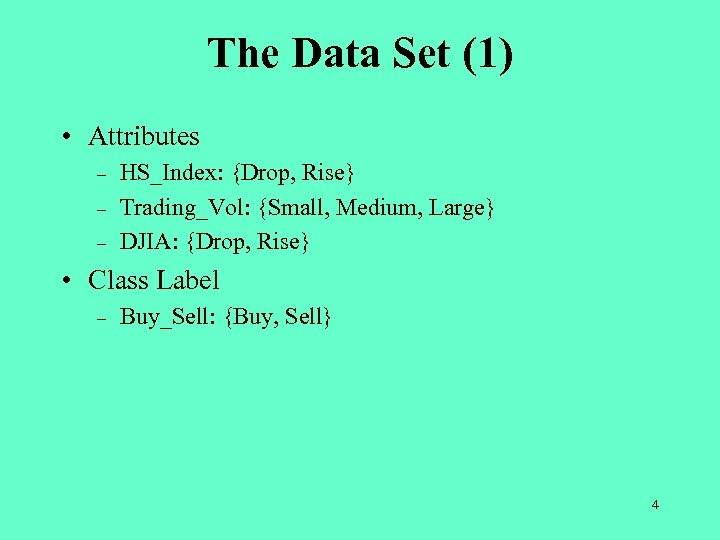The Data Set (1) • Attributes – – – HS_Index: {Drop, Rise} Trading_Vol: {Small, Medium, Large} DJIA: {Drop, Rise} • Class Label – Buy_Sell: {Buy, Sell} 4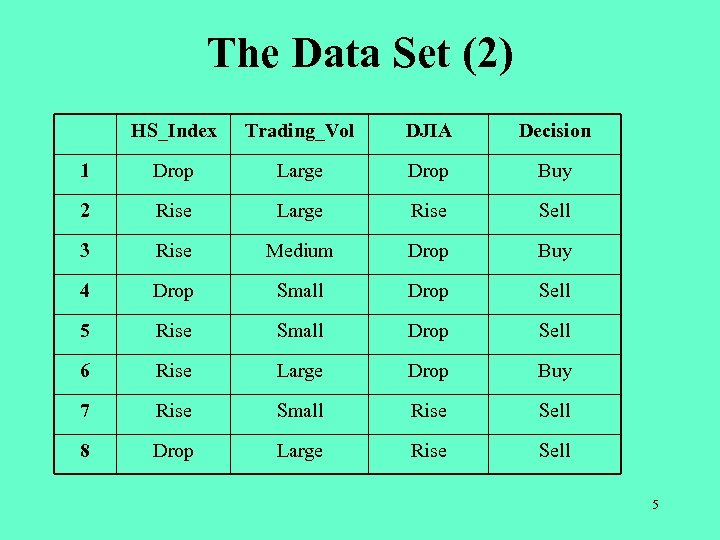The Data Set (2) HS_Index Trading_Vol DJIA Decision 1 Drop Large Drop Buy 2 Rise Large Rise Sell 3 Rise Medium Drop Buy 4 Drop Small Drop Sell 5 Rise Small Drop Sell 6 Rise Large Drop Buy 7 Rise Small Rise Sell 8 Drop Large Rise Sell 5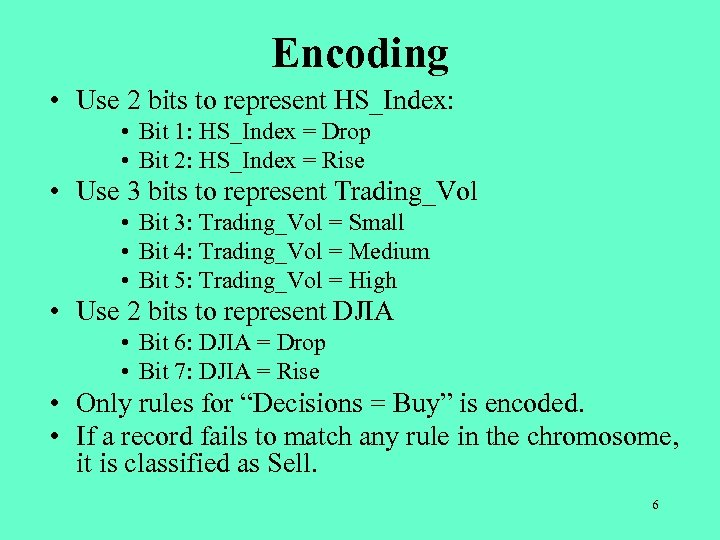Encoding • Use 2 bits to represent HS_Index: • Bit 1: HS_Index = Drop • Bit 2: HS_Index = Rise • Use 3 bits to represent Trading_Vol • Bit 3: Trading_Vol = Small • Bit 4: Trading_Vol = Medium • Bit 5: Trading_Vol = High • Use 2 bits to represent DJIA • Bit 6: DJIA = Drop • Bit 7: DJIA = Rise • Only rules for “Decisions = Buy” is encoded. • If a record fails to match any rule in the chromosome, it is classified as Sell. 6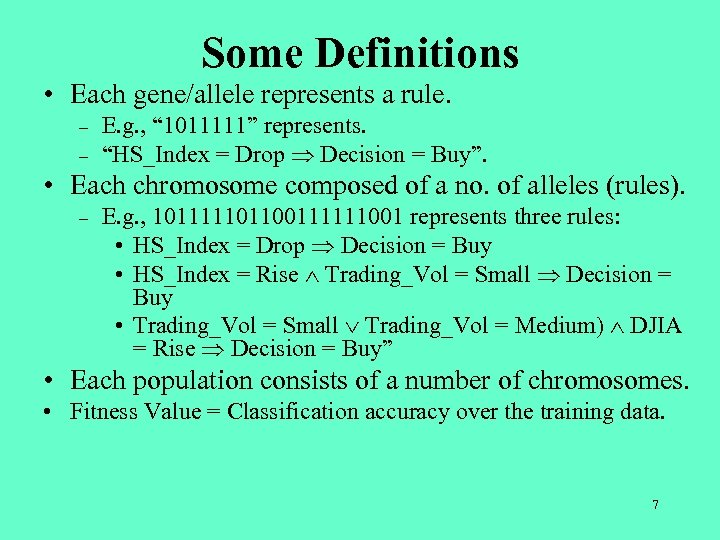Some Definitions • Each gene/allele represents a rule. – – E. g. , “ 1011111” represents. “HS_Index = Drop Decision = Buy”. • Each chromosome composed of a no. of alleles (rules). – E. g. , 101111101100111111001 represents three rules: • HS_Index = Drop Decision = Buy • HS_Index = Rise Trading_Vol = Small Decision = Buy • Trading_Vol = Small Trading_Vol = Medium) DJIA = Rise Decision = Buy” • Each population consists of a number of chromosomes. • Fitness Value = Classification accuracy over the training data. 7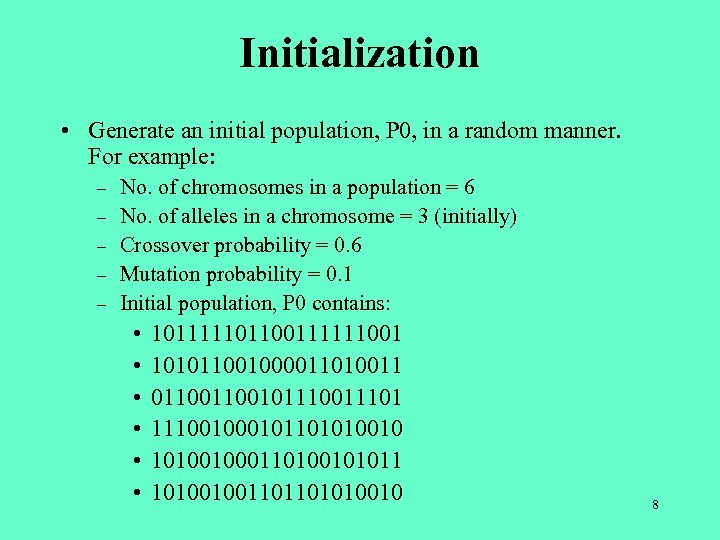Initialization • Generate an initial population, P 0, in a random manner. For example: – – – No. of chromosomes in a population = 6 No. of alleles in a chromosome = 3 (initially) Crossover probability = 0. 6 Mutation probability = 0. 1 Initial population, P 0 contains: • • • 101111101100111111001 101011001000011010011 011001011101 1110010110101001000110100101011 101001001101101010010 8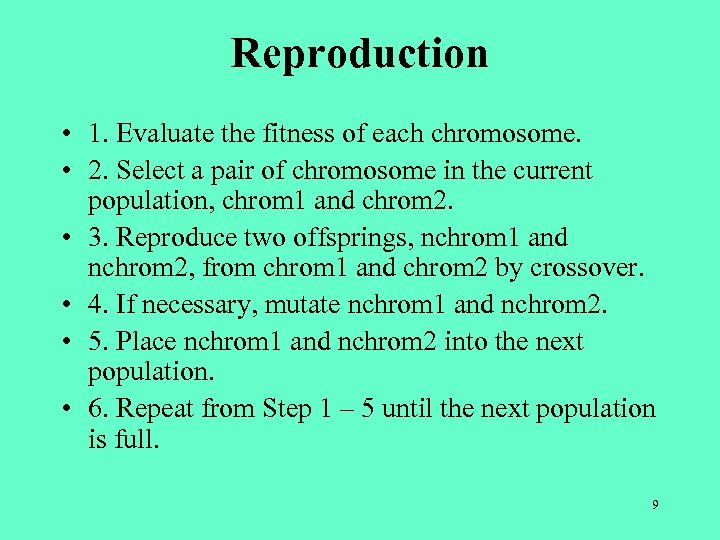Reproduction • 1. Evaluate the fitness of each chromosome. • 2. Select a pair of chromosome in the current population, chrom 1 and chrom 2. • 3. Reproduce two offsprings, nchrom 1 and nchrom 2, from chrom 1 and chrom 2 by crossover. • 4. If necessary, mutate nchrom 1 and nchrom 2. • 5. Place nchrom 1 and nchrom 2 into the next population. • 6. Repeat from Step 1 – 5 until the next population is full. 9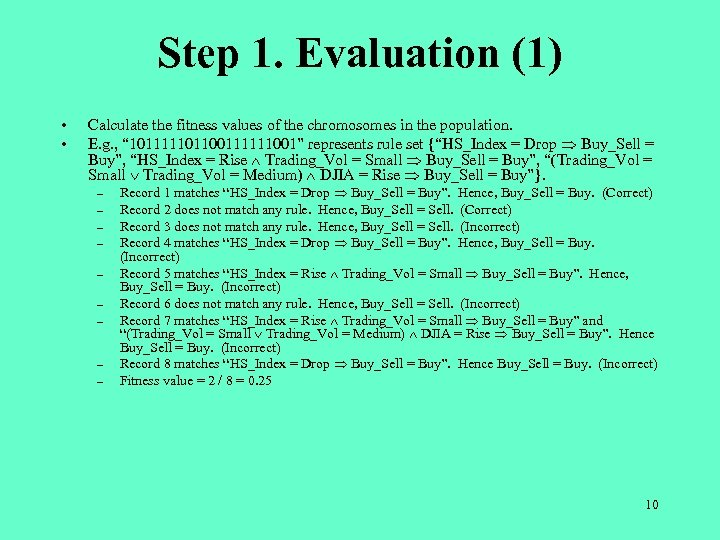Step 1. Evaluation (1) • • Calculate the fitness values of the chromosomes in the population. E. g. , “ 101111101100111111001” represents rule set {“HS_Index = Drop Buy_Sell = Buy”, “HS_Index = Rise Trading_Vol = Small Buy_Sell = Buy”, “(Trading_Vol = Small Trading_Vol = Medium) DJIA = Rise Buy_Sell = Buy”}. – – – – – Record 1 matches “HS_Index = Drop Buy_Sell = Buy”. Hence, Buy_Sell = Buy. (Correct) Record 2 does not match any rule. Hence, Buy_Sell = Sell. (Correct) Record 3 does not match any rule. Hence, Buy_Sell = Sell. (Incorrect) Record 4 matches “HS_Index = Drop Buy_Sell = Buy”. Hence, Buy_Sell = Buy. (Incorrect) Record 5 matches “HS_Index = Rise Trading_Vol = Small Buy_Sell = Buy”. Hence, Buy_Sell = Buy. (Incorrect) Record 6 does not match any rule. Hence, Buy_Sell = Sell. (Incorrect) Record 7 matches “HS_Index = Rise Trading_Vol = Small Buy_Sell = Buy” and “(Trading_Vol = Small Trading_Vol = Medium) DJIA = Rise Buy_Sell = Buy”. Hence Buy_Sell = Buy. (Incorrect) Record 8 matches “HS_Index = Drop Buy_Sell = Buy”. Hence Buy_Sell = Buy. (Incorrect) Fitness value = 2 / 8 = 0. 25 10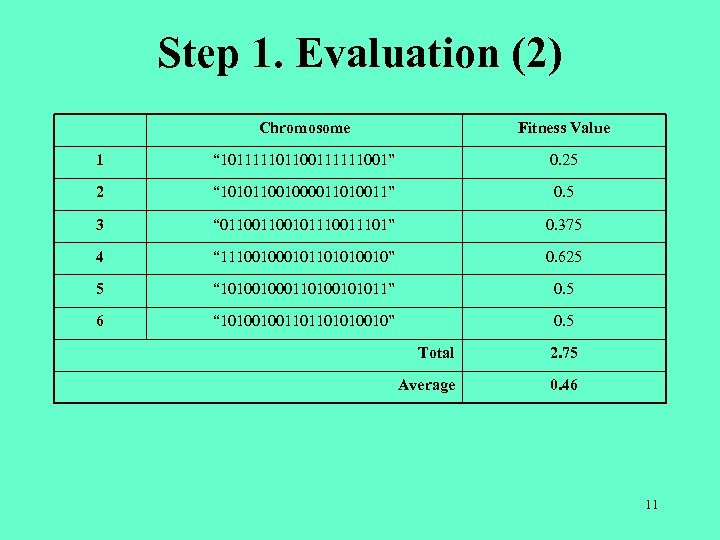Step 1. Evaluation (2) Chromosome Fitness Value 1 “ 101111101100111111001” 0. 25 2 “ 101011001000011010011” 0. 5 3 “ 011001011101” 0. 375 4 “ 11100101101010010” 0. 625 5 “ 101001000110100101011” 0. 5 6 “ 101001001101101010010” 0. 5 Total 2. 75 Average 0. 46 11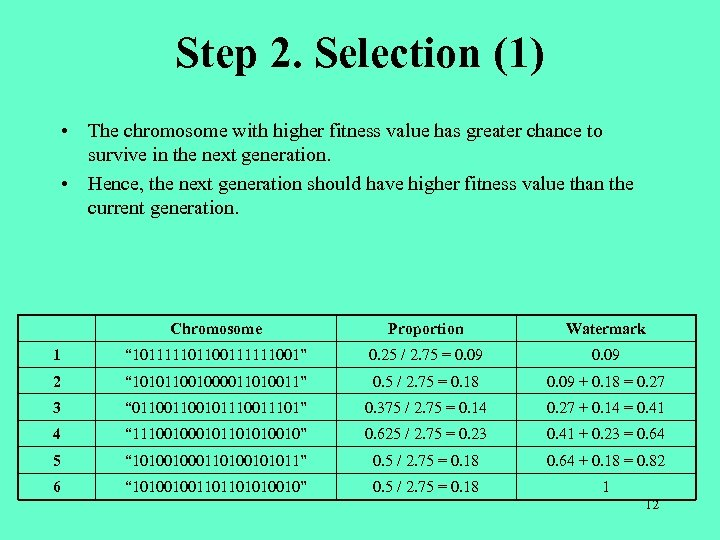Step 2. Selection (1) • The chromosome with higher fitness value has greater chance to survive in the next generation. • Hence, the next generation should have higher fitness value than the current generation. Chromosome Proportion Watermark 1 “ 101111101100111111001” 0. 25 / 2. 75 = 0. 09 2 “ 101011001000011010011” 0. 5 / 2. 75 = 0. 18 0. 09 + 0. 18 = 0. 27 3 “ 011001011101” 0. 375 / 2. 75 = 0. 14 0. 27 + 0. 14 = 0. 41 4 “ 11100101101010010” 0. 625 / 2. 75 = 0. 23 0. 41 + 0. 23 = 0. 64 5 “ 101001000110100101011” 0. 5 / 2. 75 = 0. 18 0. 64 + 0. 18 = 0. 82 6 “ 101001001101101010010” 0. 5 / 2. 75 = 0. 18 1 12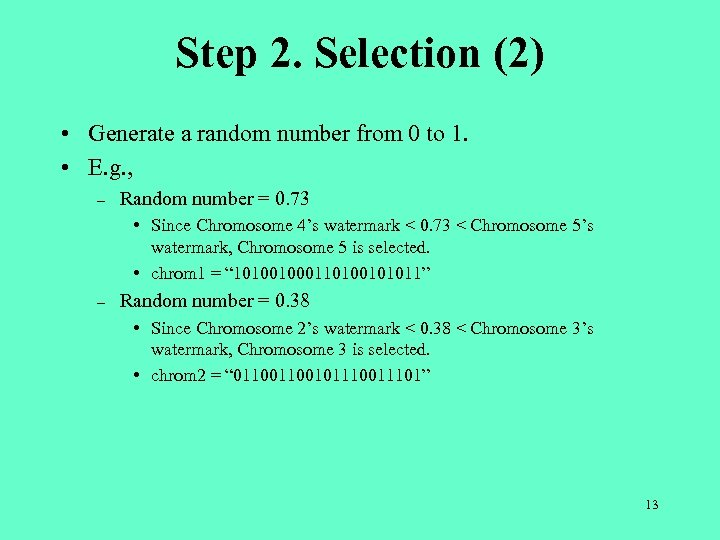Step 2. Selection (2) • Generate a random number from 0 to 1. • E. g. , – Random number = 0. 73 • Since Chromosome 4’s watermark < 0. 73 < Chromosome 5’s watermark, Chromosome 5 is selected. • chrom 1 = “ 101001000110100101011” – Random number = 0. 38 • Since Chromosome 2’s watermark < 0. 38 < Chromosome 3’s watermark, Chromosome 3 is selected. • chrom 2 = “ 011001011101” 13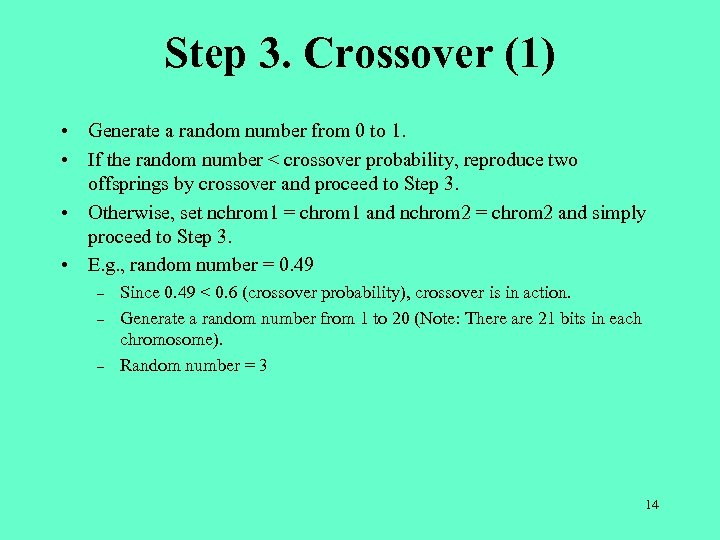Step 3. Crossover (1) • Generate a random number from 0 to 1. • If the random number < crossover probability, reproduce two offsprings by crossover and proceed to Step 3. • Otherwise, set nchrom 1 = chrom 1 and nchrom 2 = chrom 2 and simply proceed to Step 3. • E. g. , random number = 0. 49 – – – Since 0. 49 < 0. 6 (crossover probability), crossover is in action. Generate a random number from 1 to 20 (Note: There are 21 bits in each chromosome). Random number = 3 14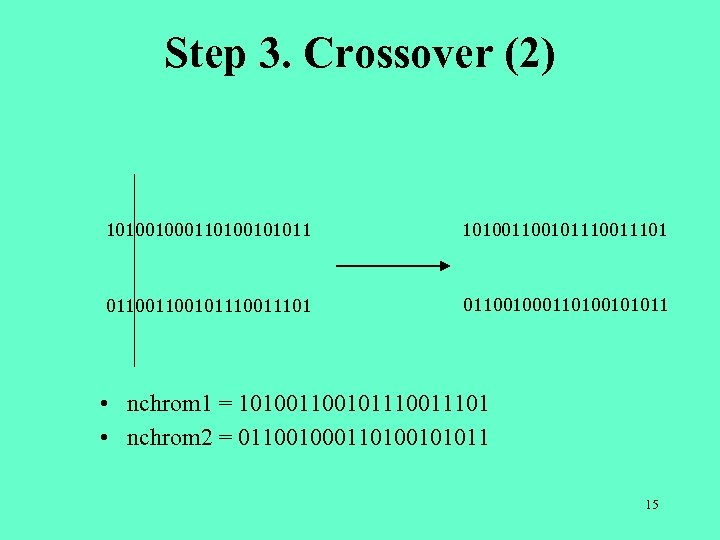Step 3. Crossover (2) 101001000110100101011 101001100101110011101 011001000110100101011 • nchrom 1 = 101001011101 • nchrom 2 = 011001000110100101011 15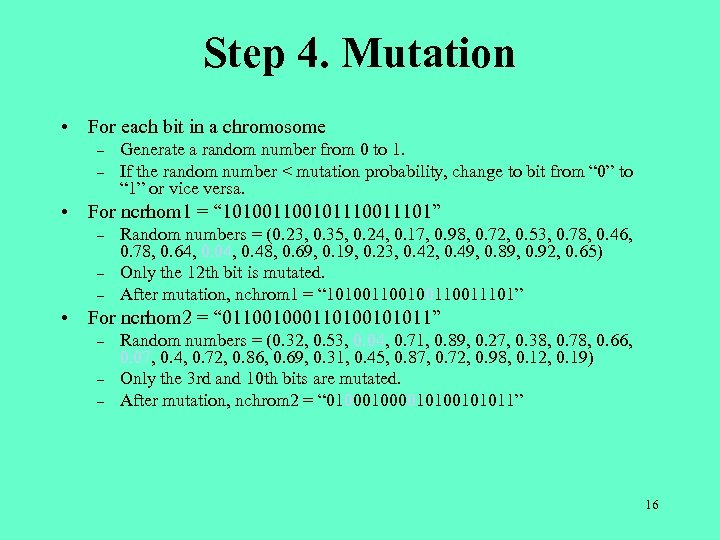Step 4. Mutation • For each bit in a chromosome – – Generate a random number from 0 to 1. If the random number < mutation probability, change to bit from “ 0” to “ 1” or vice versa. • For ncrhom 1 = “ 101001011101” – – – Random numbers = (0. 23, 0. 35, 0. 24, 0. 17, 0. 98, 0. 72, 0. 53, 0. 78, 0. 46, 0. 78, 0. 64, 0. 04, 0. 48, 0. 69, 0. 19, 0. 23, 0. 42, 0. 49, 0. 89, 0. 92, 0. 65) Only the 12 th bit is mutated. After mutation, nchrom 1 = “ 10100110011101” • For ncrhom 2 = “ 011001000110100101011” – – – Random numbers = (0. 32, 0. 53, 0. 04, 0. 71, 0. 89, 0. 27, 0. 38, 0. 78, 0. 66, 0. 07, 0. 4, 0. 72, 0. 86, 0. 69, 0. 31, 0. 45, 0. 87, 0. 72, 0. 98, 0. 12, 0. 19) Only the 3 rd and 10 th bits are mutated. After mutation, nchrom 2 = “ 010000101011” 16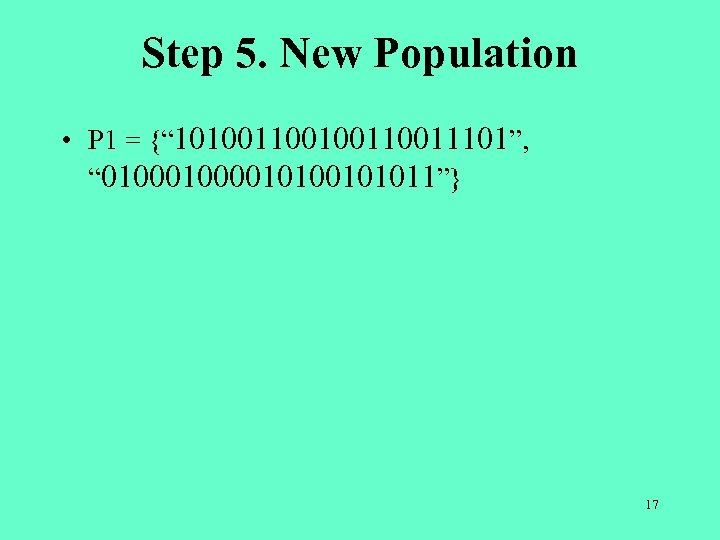Step 5. New Population • P 1 = {“ 10100110011101”, “ 010000101011”} 17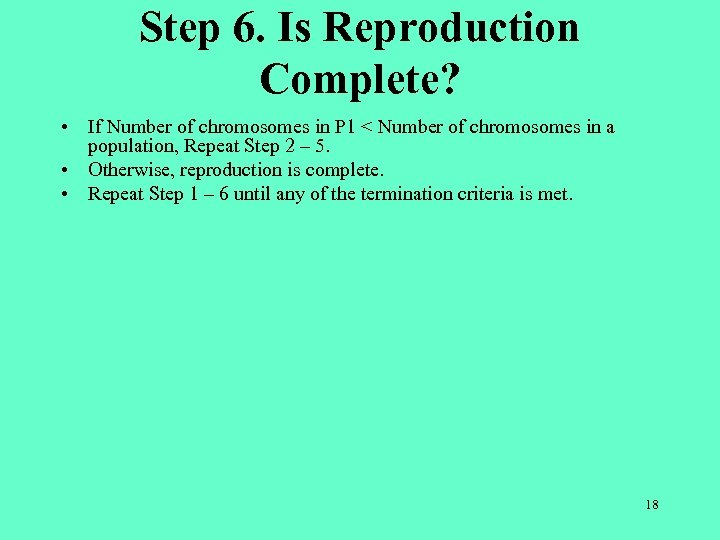Step 6. Is Reproduction Complete? • If Number of chromosomes in P 1 < Number of chromosomes in a population, Repeat Step 2 – 5. • Otherwise, reproduction is complete. • Repeat Step 1 – 6 until any of the termination criteria is met. 18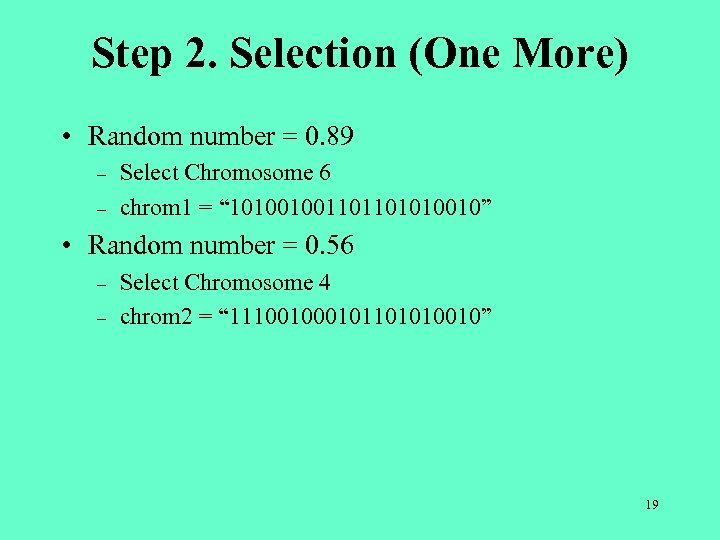Step 2. Selection (One More) • Random number = 0. 89 – – Select Chromosome 6 chrom 1 = “ 101001001101101010010” • Random number = 0. 56 – – Select Chromosome 4 chrom 2 = “ 11100101101010010” 19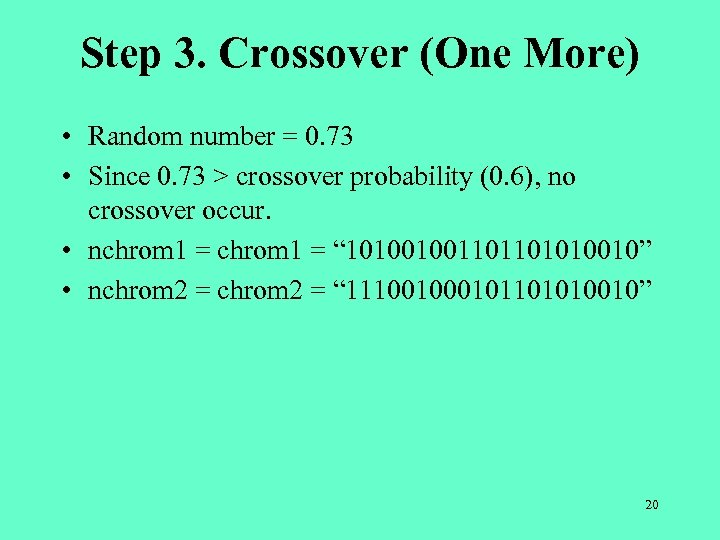Step 3. Crossover (One More) • Random number = 0. 73 • Since 0. 73 > crossover probability (0. 6), no crossover occur. • nchrom 1 = “ 101001001101101010010” • nchrom 2 = “ 11100101101010010” 20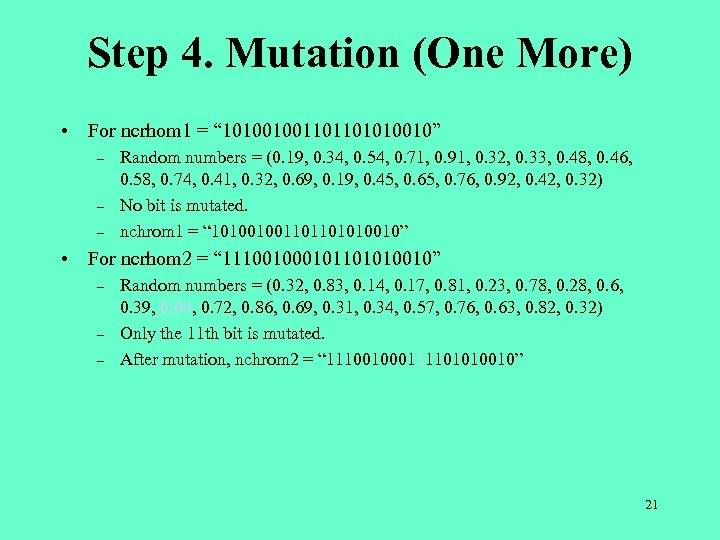Step 4. Mutation (One More) • For ncrhom 1 = “ 101001001101101010010” – – – Random numbers = (0. 19, 0. 34, 0. 54, 0. 71, 0. 91, 0. 32, 0. 33, 0. 48, 0. 46, 0. 58, 0. 74, 0. 41, 0. 32, 0. 69, 0. 19, 0. 45, 0. 65, 0. 76, 0. 92, 0. 42, 0. 32) No bit is mutated. nchrom 1 = “ 101001001101101010010” • For ncrhom 2 = “ 11100101101010010” – – – Random numbers = (0. 32, 0. 83, 0. 14, 0. 17, 0. 81, 0. 23, 0. 78, 0. 28, 0. 6, 0. 39, 0. 04, 0. 72, 0. 86, 0. 69, 0. 31, 0. 34, 0. 57, 0. 76, 0. 63, 0. 82, 0. 32) Only the 11 th bit is mutated. After mutation, nchrom 2 = “ 111001000111101010010” 21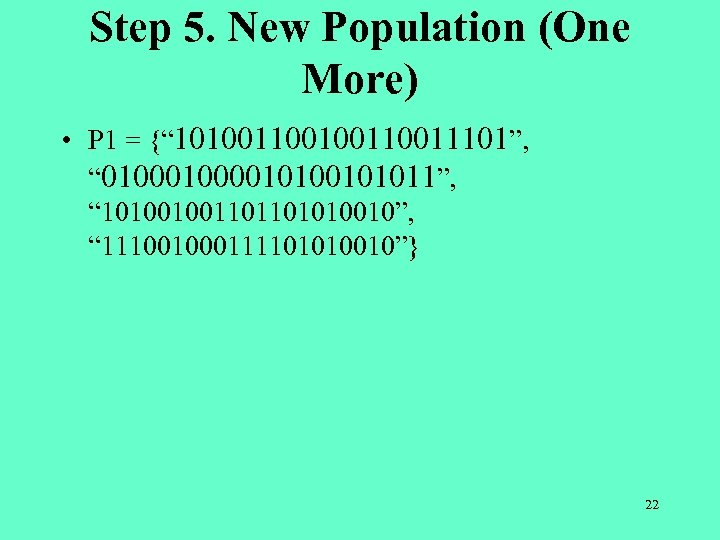Step 5. New Population (One More) • P 1 = {“ 10100110011101”, “ 010000101011”, “ 101001001101101010010”, “ 111001000111101010010”} 22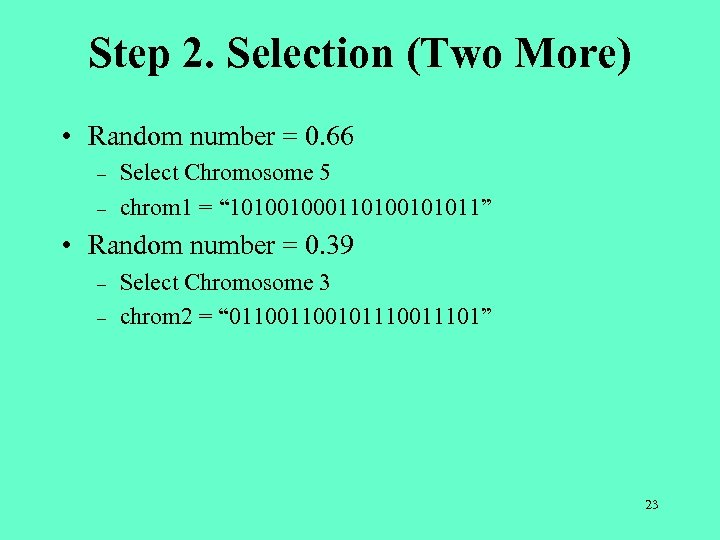Step 2. Selection (Two More) • Random number = 0. 66 – – Select Chromosome 5 chrom 1 = “ 101001000110100101011” • Random number = 0. 39 – – Select Chromosome 3 chrom 2 = “ 011001011101” 23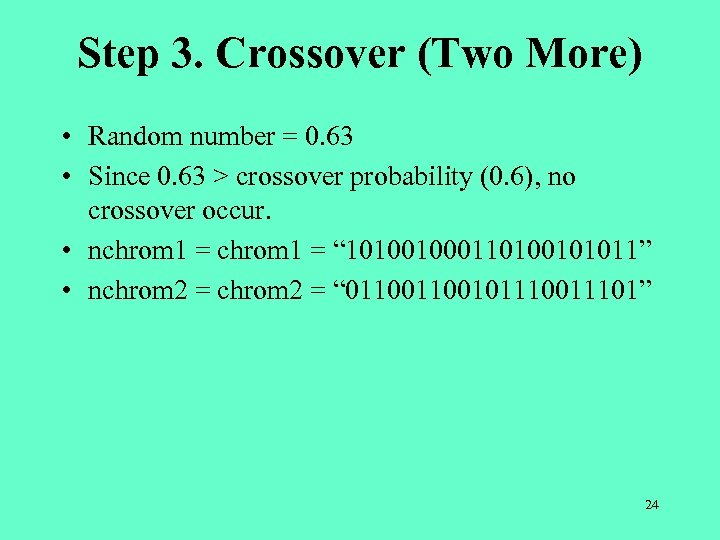Step 3. Crossover (Two More) • Random number = 0. 63 • Since 0. 63 > crossover probability (0. 6), no crossover occur. • nchrom 1 = “ 101001000110100101011” • nchrom 2 = “ 011001011101” 24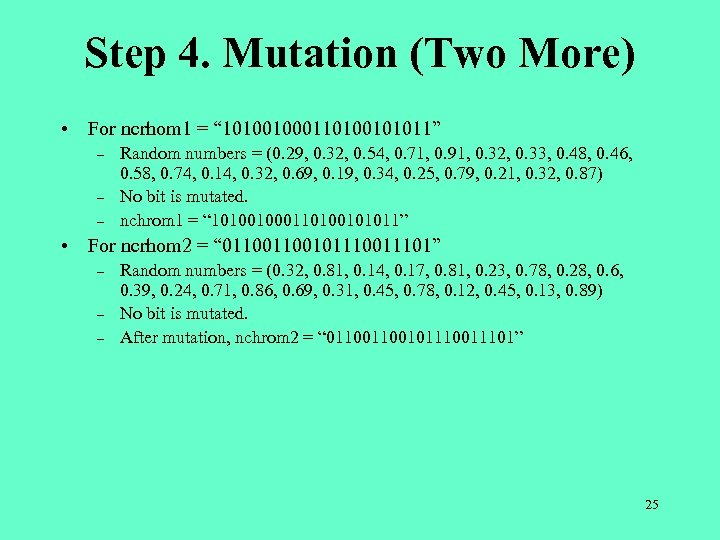Step 4. Mutation (Two More) • For ncrhom 1 = “ 101001000110100101011” – – – Random numbers = (0. 29, 0. 32, 0. 54, 0. 71, 0. 91, 0. 32, 0. 33, 0. 48, 0. 46, 0. 58, 0. 74, 0. 14, 0. 32, 0. 69, 0. 19, 0. 34, 0. 25, 0. 79, 0. 21, 0. 32, 0. 87) No bit is mutated. nchrom 1 = “ 101001000110100101011” • For ncrhom 2 = “ 011001011101” – – – Random numbers = (0. 32, 0. 81, 0. 14, 0. 17, 0. 81, 0. 23, 0. 78, 0. 28, 0. 6, 0. 39, 0. 24, 0. 71, 0. 86, 0. 69, 0. 31, 0. 45, 0. 78, 0. 12, 0. 45, 0. 13, 0. 89) No bit is mutated. After mutation, nchrom 2 = “ 011001011101” 25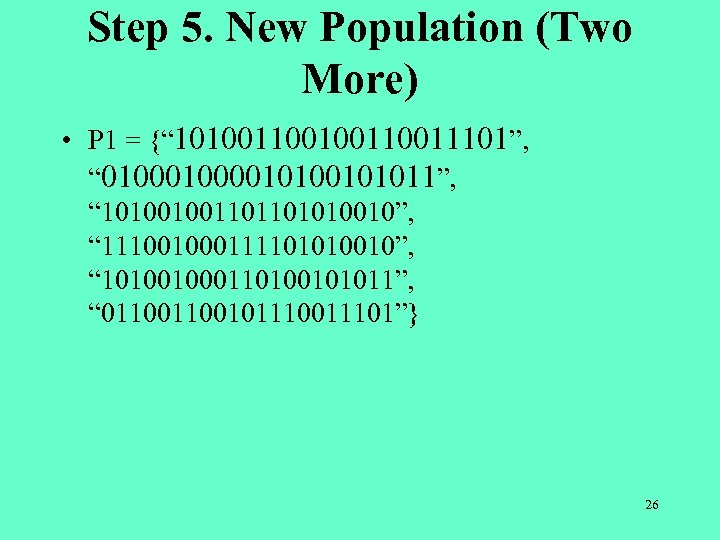Step 5. New Population (Two More) • P 1 = {“ 10100110011101”, “ 010000101011”, “ 101001001101101010010”, “ 111001000111101010010”, “ 101001000110100101011”, “ 011001011101”} 26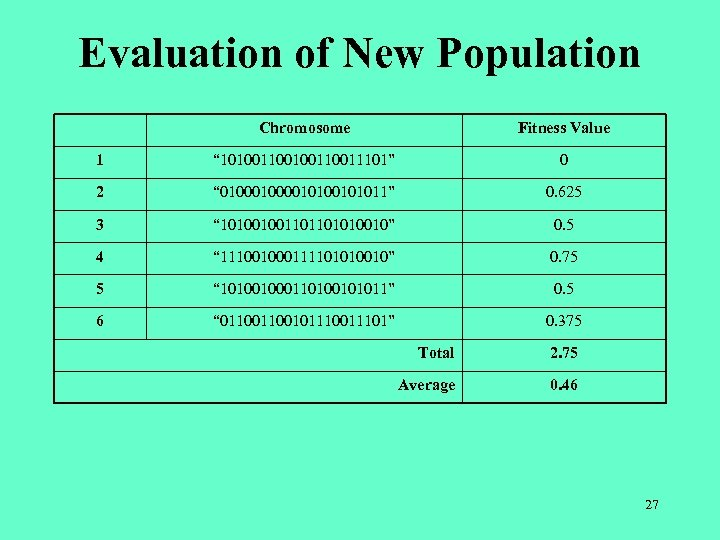Evaluation of New Population Chromosome Fitness Value 1 “ 10100110011101” 0 2 “ 010000101011” 0. 625 3 “ 101001001101101010010” 0. 5 4 “ 111001000111101010010” 0. 75 5 “ 101001000110100101011” 0. 5 6 “ 011001011101” 0. 375 Total 2. 75 Average 0. 46 27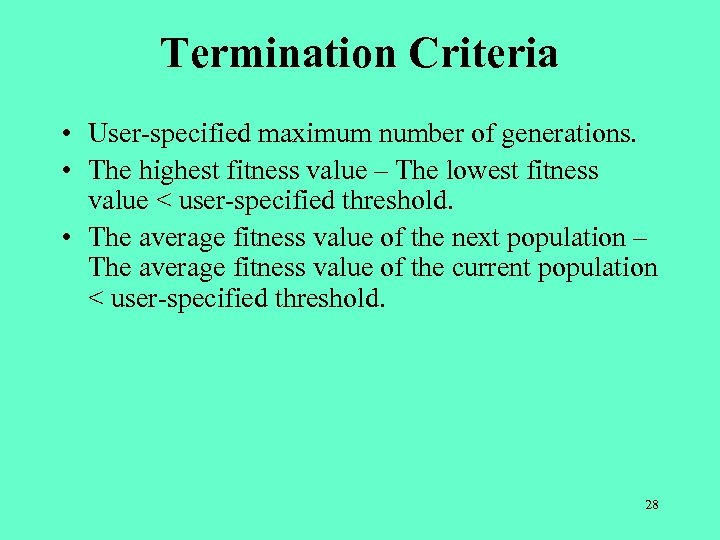Termination Criteria • User-specified maximum number of generations. • The highest fitness value – The lowest fitness value < user-specified threshold. • The average fitness value of the next population – The average fitness value of the current population < user-specified threshold. 28